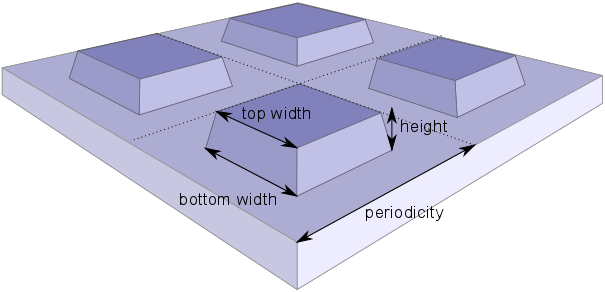# Optimization¶

In this section we present a way to efficiently optimize an optical structure. The optimization algorithm is based on a Bayesian optimization approach [Schn2017], which requires substantially fewer simulation results than other optimization algorithms [Schn2019]. As an example we revisit the structure with a square unit cell discussed in the EM-tutorial. The goal of the optimization is to create a silicon metasurface with a minimal reflectivity, which can act as an antireflective coating. The design and fabrication of such a coating has been discussed in [Prou2016].

We consider a periodic grating with square-like structures. The variable parameters of the system are shown in the figure below.The metasurface is parametrized by the periodicity, the height of the etched structures, their bottom, and their top widths.

First, we define the search domain for the minimization of the reflectivity:

```domain = {};
domain(1).name = 'height';
domain(1).domain = [50,200];
domain(2).name = 'width_bottom';
domain(2).domain = [60,200];
domain(3).name = 'width_top';
domain(3).domain = [60,200];
domain(4).name = 'periodicity';
domain(4).domain = [150,400];
```

The bottom and top widths may not exceed the periodicity of the structure. Hence, we have to define two constraints of the search domain. A constraint is defined by a function expression that returns a negative value, if and only if the constraint is fulfilled.

```constraints = {};
constraints(1).name = 'max_width_bottom';
constraints(1).constraint = 'width_bottom - periodicity + 10';
constraints(2).name = 'max_width_top';
constraints(2).constraint = 'width_top - periodicity + 10';
```

With the `domain` and `constraints` variables one can now create a new `study` object. We set the maximal number of optimization iterations to 20 and the number of parallel evaluations of the objective function to 2.

```study = jcmwave_optimizer_create_study('domain', domain, 'constraints',constraints, ...
'name','Optimization of anti-reflection grating');
study.set_parameters('max_iter', 20, 'num_parallel', 2);
```

In order to perform parallel evaluations of the objective function, we start up the daemon. Details on how to use the daemon are described in the tutorial Parallel Parameter Scan.

```jcmwave_daemon_startup();
'Multiplicity', 2, ...
```

The objective is to minimize the average reflectivity for multiple wavelengths

```lambdas = [600 700 800];
permittivities = [15.590 + 0.21635j 14.317 + 0.092101j 13.646 + 0.048345j];
```

This is the minimization loop, that is described in more detail down below:

 ``` 1 2 3 4 5 6 7 8 9 10 11``` ```while(not(study.is_done)) suggestion = study.get_suggestion(); job_ids = start_jobs(suggestion.sample, lambdas, permittivities); study.open_jobs(job_ids, suggestion); if(study.num_open_suggestions == study.num_parallel) % wait and gather results until an observation can be added [sug_results, sug_id] = study.gather_results(); obs = reflectivity(sug_results, lambdas, permittivities, domain); study.add_observation(obs, sug_id); end end ```

In order to parallelize the computation, we split up the computation of the objective function in two parts:

1. As long as the study is not finished (`not(study.is_done())`) we retrieve new suggestions (line 2). We define a function `start_jobs` that starts for each suggested parameter set 3 scattering computations (one for each wavelength) and returns the corresponding job ids (line 3) [*].

```function job_ids = start_jobs(sample, lambdas, permittivities)
job_ids = [];
keys = sample;
keys.slc = 150;
for ii = 1 : numel(lambdas)
keys.lambda0 = lambdas(ii)*1e-9;
keys.permittivity = permittivities(ii);
job_id = jcmwave_solve('project.jcmp', keys, 'temporary', 'yes');
job_ids(end+1) = job_id;
end
end
```

The job ids are associated with a suggestion by calling `study.open_jobs(job_ids, suggestion)` (line 4).

2. Since we set `num_parallel=2`, we can retrieve only two parallel suggestions and start in total 6 parallel jobs. Afterwards (line 5), we have to add an observation to the study. To this end, we call `[sug_results, sug_id] = study.gather_results();` (line7). This function waits until all jobs of any of the open suggestions have finished and returns the corresponding cell array of results and the suggestion id. Based on these results, we can finally compute the reflectivity by defining a function `reflectivity()`

```function obs = reflectivity(results, lambdas, permittivities, domain)
refl = 0;
derivatives = zeros(numel(domain),1);
pref = 1/numel(lambdas);
for ii = 1 : numel(lambdas)
FC = results{ii}{2}.ElectricFieldStrength{1};
this_refl = sum(real(FC).^2 + imag(FC).^2);
refl = refl + pref*this_refl;
for jj = 1 : numel(domain)
dfT = getfield(results{ii}{2},['d_' domain(jj).name]);
dFC = dfT.ElectricFieldStrength{1};
d_this_refl = sum(2*(real(FC).*real(dFC) + imag(FC).*imag(dFC)));
derivatives(jj) = derivatives(jj) + pref*d_this_refl;
end
end

obs = Observation();
deriv_vecs = eye(numel(domain));
for ii = 1 : numel(domain)
end

end
```
The convergence of the optimization can be largely improved by providing also derivative information. To this end the derivatives of the Fourier coefficients are calculated by the solver as described in this tutorial example . The objective function returns an `observation` object containing the objective value and its derivatives. We add the observation to the study by calling `study.add_observation(obs, sug_id);` (line 10).

The progress of the optimization can be examined in a dashboard, that is shown in a browser window. A typical example is shown in the figure below. By optimizing the parameters one can reduce the reflectivity from 30% to below 1%.

Input Files

 [*] To speed up the computation times for this tutorial example, we set the side length constraint of the mesh to 150nm (`keys['slc']=150`). In order to obtain converged results, one should rather use a value of 40nm.
 [Schn2019] P.-I. Schneider, X. Garcia Santiago, V. Soltwisch, M. Hammerschmidt, S. Burger, C. Rockstuhl. Benchmarking Five Global Optimization Approaches for Nano-optical Shape Optimization and Parameter Reconstruction ACS Photonics 6, 2726 (2019) https://doi.org/10.1021/acsphotonics.9b00706 https://arxiv.org/abs/1809.06674
 [Schn2017] P.-I. Schneider, X. Garcia Santiago, C. Rockstuhl, S. Burger. Global optimization of complex optical structures using Bayesian optimization based on Gaussian processes Proc. SPIE 10335 (2017) 103350O (2017) https://doi.org/10.1117/12.2270609 https://arxiv.org/abs/1707.08479
 [Prou2016] Julien Proust, Anne-Laure Fehrembach, Frédéric Bedu, Igor Ozerov and Nicolas Bonod. Optimized 2D array of thin silicon pillars for efficient antireflective coatings in the visible spectrum. Scientific Reports volume 6, Article number: 24947 (2016) http://dx.doi.org/10.1038/srep24947